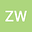•This paper considers the initial value problem for a normal hyperbolic curvature flow derived by the cell-based mathematical models of tissue growth to account for the mechanistic influence of curvature on cell evolution. The equations satisfied by support functions under this flow is a hyperbolic Monge-Amp$\grave{e}$re equation. The equation for both perimeter and area of closed curves under the flow are also obtained. Based on this, we show that for a closed curve, if the initial velocity $v_{0}<0$, the solution of this flow converges to a point in finite time; if $v_{0}>0$, the solution of this flow exists for all $t\in[0,\infty)$.